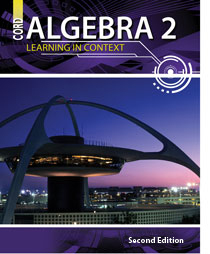# Algebra 2 - 2nd Edition

## Chapter 9: Sequences and Series

Some links are repeated for use with more than one lesson.

### 9.1 Patterns

http://www.learner.org/teacherslab/math/patterns/buttons/

http://www.mathman.biz/html/patterns.html

http://nlvm.usu.edu/en/nav/frames_asid_171_g_4_t_2.html?open=
activities&from=category_g_4_t_2.html

This page contains an applet using geometric shape to create patterns. This website requires Java to run some of its features.

### 9.2 Arithmetic Sequences and Series

http://hotmath.com/help/gt/genericalg2/section_9_2.html
This page provides several practice problems that work with arithmetic sequences and series.

http://www.mathsisfun.com/algebra/sequences-sums-arithmetic.html
This page explains how to define and sum an arithmetic series, including practice problems.

### 9.3 Geometric Sequences and Series

mathlab/col_algebra/col_alg_tut54d_geom.htm

http://www.algebralab.com/practice/practice.aspx?
file=Algebra_GeometricSequencesSeries.xml

### 9.4 Infinite Geometric Series

http://www.purplemath.com/modules/series.htm
This series of pages is a tutorial with many examples of series and sequences for this chapter, concluding with infinite geometric series for this lesson.

http://www.mathsisfun.com/algebra/sequences-sums-geometric.html
This page provides many examples of geometric series, concluding with infinite geometric series.

http://www.coolmath.com/fractals/gallery.htm
This page includes a link to a lesson on fractals, a gallery of many colorful fractals, and a fractal generator to make your own fractal art.

### 9.5 The Binomial Theorem

http://www.intmath.com/Series-binomial-theorem/4_Binomial-theorem.php